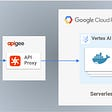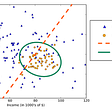# Learning Machines With Me. (Part Two)

A series where I attempt to not only understand but also do fun things with machine learning — with strangers on the Internet.While the cool kids are playing football with their feet, we’re going to play football on spreadsheets

Welcome back! If this is the first time you’ve come upon this series, please check out the first article in the series by clicking me. There we discussed regression and used it to build a model that could predict a team’s position based on basic information.

In today’s session, we’re going to get into classification by building a model that can classify a player’s position based on information about a player. All very cool stuff, I know! So let’s get right into it.

Just like before, here is how to approach this guide:

All you have to do is learn some of the basic level things that are occurring and then, download the dataset and simply run the code! After that, feel free to play around with the code as you see fit and try to make a better model!

# In this second part of the series, we’re going to understand classification — the second building block in a beginner’s attempt to understand machine learning.

Classification is as the name sounds — simply put, given some information about certain objects, we’re trying to classify — label — these objects. Classification, and regression, falls under a category of machine learning called supervised learning.

In supervised learning, the machine actually is being guided — supervised — by the input to which we already know the answers. In other words, we actually already know how a certain input will look like and we use those examples to train the model.

As such, classification is supervised learning because well, the machine needs to know what/how a certain label looks like/is before it attempts to label right? The same way you need to actually see how a great dribbler is to then label great/not-great dribblers, the machine needs that information so it can label.

Other than that, that’s simply it to classification. Now, there are multiple classification models that one can construct. Here are some:

• Logistic Regression (don’t judge a book by its cover!)
• K Nearest Neighbours
• Support Vector Machine Classification
• Kernel SVM
• Naive Bayes Classification
• Decision Tree Classification
• Random Forest Classification

For this model, we’re going to go with a Naive Bayes Model. Why this one?

Let’s get into the specifics of what goes in this model.

The Naive Bayes Model works its whole classification system based on a theorem in probability called: Bayes Theorem.

You might have seen it if you’ve taken a stat class. Here’s how the theorem looks like:

You’re probably wondering, what the hell does this even mean?!

Let’s break all four parts of the components down one-by-one with an example scenario.

Let’s say we have a pool of 100 players and 40 play in La Liga while 60 play in the Premier League. Let’s say, then that out of all 100 players, 20 players are strikers (just go with me).

Out of those 20 strikers, 15 are from Premier League while 5 are from La Liga.

Now, let’s say we want to find the probability that a player is a striker given that the player is from the Premier League.

Looking at numbers, this is easy. The answer is 15/60 (player is a striker that is in PL / player is from PL).

Now let’s get a generalized formula for this. A more generalized version allows us to work large datasets where we might only percentages/probabilities.

• P(B) : This is the probability that a player is from the PL — 60%
• P(A) : This is the probability that a player is a striker — 20%
• P(B | A) : This is called a conditional probability and the way you read this is: “What’s the probability of me getting B GIVEN that I have A”. So here, what is the probability that a player is from a PL given that we have a striker? Well we know that out of 20 strikers, 15 are from PL. As such, we get 75%.
• P(A | B) : This looks similar to what we just did but is not the same! This reads: “What’s the probability of me getting A GIVEN that I have B”. So here, what is the probability that a player is a striker given that we have a player from the PL? This is what we want to know.

Let’s apply the formula:

P(A | B) = (0.75 * 0.20) / (0.60) = 0.25 = 25%

Okay, so basically Bayes allows a strong method of determining probabilities. How can we use this in classification?

Well, what are we trying to do in classification? Let’s look back to what we defined classification as:

given some information about certain objects, we’re trying to classify — label — these objects.

Is this not the same as finding the probability an object belongs to a label based on some information about that object?

If we do this for both two labels, we can compare the probabilities — given by the Bayes Theorem — and whichever one has a higher probability is the label we should assign to the object.

And that is what the Naive Bayes Model attempts to do.

# Measuring Accuracy

Ok, now let’s say we’ve made a classification model. How do we determine its accuracy?

Well, for starters we can just see the classifications we got right divided by the total observations.

But this is a trap!

To see how, we’re going to construct something called a confusion matrix (it’s not confusing, trust me).

Simply put, we compare our predicted labels against our actual labels. We get to see where we got things correct and where we got it right.

Let’s put some numbers in there to see how accuracy rate can be troubling.

Let’s say our model comes up with these matrix where the numbers tell how many observations (ex: we got 100 rows right where the label was 0 and we predicted 0 as well).

Here, our accuracy rate is: 140/200 or 70%

What if we can better our accuracy rate but make a worse model? Sounds impossible, yeah? What if we just assigned EVERYTHING to 0. Here’s what happens:

Now our accuracy rate is 150/200 or 75%.

We just got a higher accuracy rate while making a worse model! As such, to better classify how good classification models, we use a combination of confusion matrix and accuracy rate.

When we build our confusion matrix, keep an eye here:

Bottom-right are the players we predicted to be in our position that were actually that position. Upper-left are players we predicted NOT to be in our position that were actually NOT in that position.

Naturally, the upper-left number will be greater than the bottom-right number but ensure that the bottom-right number doesn’t go to zero!

With that out of the way, let’s go to the dataset and code!

# Building Our Classification Model

I have compiled datasets from the EPL and LaLiga seasons of 2020/21 — this is our training dataset. In addition, I have compiled a dataset of Dortmund’s season of 2019/20 to help us build this model — this is our test dataset.

For our purposes, we want to classify and label left-backs (but later down, I talk about how you can change the code to classify other positions).

In this dataset, I have compiled the average starting points (x and y) and average ending points (x and y) of players’ passes and, in addition, the convex hull area (a representation of the area of a player’s touch density). These are basic features for a player and we can always create new metrics to improve the precision but since we’re building a basic version, these should suffice.

In addition, the training dataset contains identifiers for whether a player is a left-back or not. After training our model on the training dataset, we’ll apply it on Dortmund’s dataset to see how good our model was.

Here is the link to the Python notebook: Code for the Model

Here is the link to the Training Dataset: TrainingDataset

Here is the link to the Test dataset: TestDataset

Here are some instructions for running this:

• Download the dataset and store it somewhere (remember where you store this!)
• Make a copy of the Notebook so that you can edit and import the data!
• Click this Folder option:
• Here, find the dataset on your computer/laptop and upload it. (Note: this dataset will only exist for this Notebook while you have the Notebook open. If you close the Notebook and restart it, you will have to reupload the dataset again!)
• Now, you can either run all the cells at once OR run it one by one if you make some changes.
• Clicking Run-All runs all cells. Clicking the brackets ( a play button will appear ) will only run that cell.

At the end of the notebook, you’ll see the classification model working on the testing set with its indications of what is a left-back (1) and what is not a left-back (0).

# Some Interactivity:

Now, this series is meant to be interactive so how do you make your own models/improve/change things?

Changing/implementing these features/functions will result in different accuracy scores at the end which helps you determine whether the model for certain labels is good/bad.

One thing is to change the type of classification model that you use:

Try implementing the simplest classification model — logistic regression:

`from sklearn.linear_model import LogisticRegressionclassifier = LogisticRegression()classifier.fit(X_train, y_train)`

Try classifying positions other than left-backs! (This is where the fun really is!)

To do this, we need to change our training dataset as we need to update our labels to indicate the position we pick.

These are some of the positions my training dataset has to offer:

`DC, DL, DR, MC, FW, AMC, MR, DMC, ML`

Pick one and then, before splitting the dataset into training/testing, change this piece of code:

`dataset = pd.read_csv('TrainingDataset.csv',encoding='latin-1')dataset['identifier']= np.where(dataset['pos']=="INSERT POSITION PICKED", 1, 0)X = dataset.iloc[:, 1:-2].valuesy = dataset.iloc[:, -2].values`

From there, run the model as you did before! See the differences, see if our original model works on different positions, see if the logisitic regression works better for different positions — explore! Some positions do not offer great results (mainly very niche positions like AMC, etc) but others like DC and DL offer accurate results!

Remember, this is a basic model based on the most basic statistics. See if adding data from FBref can maybe increase our classifications!

Please feel free to reach out to me on Twitter if you have any questions. Leave any comments on how to improve!

--

--

--

## More from Abhishek Amol Mishra

Writer. Aspiring Computer & Data Scientist. “We’re made of star stuff. We are a way for the cosmos to know itself “ — Carl Sagan

Love podcasts or audiobooks? Learn on the go with our new app.

## Serverless Prediction at Scale, Part 2: Custom Container Deployment on Vertex AI## Dog Breed Classification — Udacity Capstone## Understanding Objectness in Object Detection Models## Which ML Algorithm to Use ?## Deploying a Deep Learning pipeline with Docker on AWS## Does Ensemble Models Always Improve Accuracy?## Using Computer Vision to Identify Critical Anatomy in Surgical Images## How to Deploy Machine Learning Models using Flask, Docker and Google Cloud Platform(GCP)## Abhishek Amol Mishra

Writer. Aspiring Computer & Data Scientist. “We’re made of star stuff. We are a way for the cosmos to know itself “ — Carl Sagan

## Football Like Never Before## Using Deep Stats for Performance-Based Soccer Player Valuations## AFCON 2021: A Complete Guide